# Sentiment Wigi: Closing Score with Volume Kick

Dilihat 2536 kali
2536
One of the great advantages to TradingView is the opportunity to see the market through many different individual’s unique perspectives. Each new perspective offers the opportunity to gain some additional insight and thus advantage. The concept being explored in this indicator is the disclosure of the sentiment of the traders in control of the current price action. If we can accurately determine trader’s sentiment, we can determine where the market will go next. Maybe not tomorrow, but eventually.

The Range of the day, sometimes referred to as the “True Range” is divided into 100 equal segments. You can then determine a Closing Score for the close of the day by determining where on this range, 0 – 100, the closings occurred. The pine script code for that calculation is: CS100 = (((close-low) / (high-low) *100.

Anything above 70 indicates a strong close and positive trader sentiment. A close around 100 or very close to it, is so strong that it can actually indicate the end of a run, and thus has the potential to be a contrarian indicator.

Any close below 30 indicates a weak close and negative trader sentiment. Close’s, close to or at zero indicate very negative sentiment and just as a close at 100 can be counter intuitive, a close at zero often indicates exhaustion within the traders of this equity, and you can see prices climb from this point forward about 50% of the time.

The negative close scores (less than 50) are inverted to produce the same length of the bars as the positive scores (greater than 50).

Inputs for this indicator, “UPPER CLOSING SCORE TRIGGER” and “LOWER CLOSING SCORE TRIGGER” allow for the user to determine where positive and negative signals are cutoff. I set them at 70 for the positive indicator and 30 for the negative indicator. Neutral numbers, those ranging between 31 and 69 do not provide enough certainty to help assist in your trading decisions.

I consider volume to be the second most important feedback from the markets that we, as traders have the opportunity to view, second only to price. For this reason the only columns that are drawn is when the volume is greater than the Average Daily volume ( ADV ). This is a very simple calculation (VxCNT = (V/VOLMA) and provides a factor that the Closing Score is multiplied by. Thus, each column drawn on the chart displays the Closing Score multiplied by a volume factor that is obtained by dividing the Volume Mov Avg by todays Volume . There is also an input to adjust this parameter as well and is titled “EMA Length To Calculate Lookback.”

If you want to liberalize the threshold at which volume is considered you can accomplish that in two ways. Change the length of the VOLMA in inputs and adjust the input titled “VOLTEST.” VOLTEST is currently set at (1) and this produces a simple test: is todays Volume above the current Vol Mov Avg? Any number less than 1 such as 0.95 would allow you to consider volumes that are close to, but not above the current Vol Mov Avg .

The Closing Score is plotted as Columns with the longer bar behind it providing the added strength that you can infer from that Vol of that day.

Soon I hope we will be able to scan for stocks meeting specific conditions. Here is what I will be scanning for using this indicator. When you see several strong green bars as a stock’s price is declining, you have a good candidate to watch closely to see a strong reversal. This indicator is also very helpful when trying to determine which way a stock will break when at a decisive point of any chart pattern, such as an ascending triangle .

Small boxes along the blue zero line indicates if any of the following three conditions have appeared. White boxes indicates Dojis, Red boxes indicates a close, very close to zero and a green box indicates the close was close to 100.
study("CSxVol")
// • INPUTS & CONSTANTS
C = (close), H = (high), L = (low), O = (open), V = (volume)
MALength       = input (7,        title="EMA Length To Calculate Lookback")
CS100TT        = input (50,       title="UPPER CLOSING SCORE TRIGGER")
CS100BT        = input (50,       title="LOWER CLOSING SCORE TRIGGER")
VOLTEST        = input (1,        title="POSITIVE OR NEG VOL TEST RESULT:  >1 = POSITIVE, < 1 + NEGATIVE")
VOLMA    = ema(V, MALength)                 // EXP MOV AVG of VOLUME
VxCNT = (V/VOLMA)                           // % DIF of VOL from VOL MOV AVG
CS100  = (((C-L) / (H-L) *100))             // CLOSING SCORE CALCULATION 0 - 100
CSA50VT  = ((CS100 >= CS100TT)  and (VxCNT > VOLTEST)) ? ((CS100)* VxCNT ):na
CSB50VT  = ((CS100 <= CS100BT)  and (VxCNT > VOLTEST)) ? ((abs(CS100 -100)*-1)* VxCNT ):na
CSA50  = ((CS100 >= CS100TT) and (VxCNT > VOLTEST)) ? ((CS100)):na
CSB50  = ((CS100 <= CS100BT) and (VxCNT > VOLTEST)) ? ((abs(CS100 -100)*-1)):na

// DETERMIN IF CLOSE EQUALS ZERO OR 100
CLOSEFLAGa100 = (CS100 >= 97) ? (10):na
CLOSEFLAGb100 = (CS100 >= 97) ? (-10):na
CLOSEFLAGa0   = (CS100 <= 3) ? (10):na
CLOSEFLAGb0   = (CS100 <= 3) ? (-10):na
// • DOJI
DOJIX = (abs(O-C)<=((H-L)*0.1))
DOJIT = DOJIX ? 10 : DOJIX >=1
DOJIB = DOJIX ? -10 : DOJIX >=1
// HLINES PLOTS

hline(0, title="Zero", color=blue, linestyle=dotted, linewidth = 2)
// • PLOTS
// TOP PLOTS
plot(CSA50VT,         title= "CS > 50", style=columns, color=#00FF00,   linewidth=4, transp = 50)
plot(CSA50,         title= "CS > 50", style=columns, color=#00FF00,   linewidth=4, transp = 50)
// BOT PLOTS
plot(CSB50VT,         title= "CS < 50", style=columns, color=red,   linewidth=4, transp = 50)
plot(CSB50,         title= "CS < 50", style=columns, color=red,   linewidth=4, transp = 50)
// • DOJI PLOTS
plot(DOJIT,         title= "DOJI TOP",              style=columns,  color=white,    transp=10)
plot(DOJIB,         title= "DOJI BOTTOM",           style=columns,  color=white,    transp=10)
plot(CLOSEFLAGa100, title= "CS100 TOP",             style=columns,  color=#00FF00,    transp=10)
plot(CLOSEFLAGb100, title= "CS100 BOTTOM",          style=columns,  color=#00FF00,    transp=10)
plot(CLOSEFLAGa0,   title= "CS0 TOP",               style=columns,  color=red,      transp=10)
plot(CLOSEFLAGb0,   title= "CS0 BOTTOM",            style=columns,  color=red,      transp=10)
plot(CS100,         title= "DOJI TOP",              style=columns,  color=#FFC125,   transp=100) // Set at Zero Transparancy so arguments appear at top of indicator
plot(VxCNT,         title= "DOJI TOP",              style=columns,  color=aqua,     transp=100) // Set at Zero Transparancy so arguments appear at top of indicator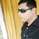TRULY AMAZING WORK SIR THOUGH I HAVE STUDIED 1000'S INDICATORS INCLUDING ARTIFICIAL NEURAL NETWORK THIS THE STRATEGY I WAS LOOKING FOR YOU HAVE MADE MY DAY TODAY AS IT IS SIMPLE AND CAN BE USED BY MANY TRADERS WHO ARE NOT EXPERT ....MY STANDING SALUTE TO U FOR THIS AMAZING BRAIN & INTELLIGENCE THAT YOU HAVE ...YOU ARE NOT ONLY SUPER STAR BUT ROCK STAR ....BLESSING AND PROSPER FOREVER ...REGARDS FROM INDIA ...FOLLOWING YOU
Balas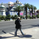From Volume Spread Analysis point of view, thi indicator is very helpful. Thanks.
Balas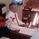Very helpful. Each layer of information should complement each other, as yours does (CS & L Tails CNT). Almost like looking over the shoulder of big money:)
Balas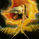What is the meaning of the 3 colors; white, yellow/gold, cyan for doji top?
Thank you for these indicators!
Balas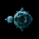Thanks for this. Thanks for sharing the calculations and how they are derived and the explanation behind the meaning. Too often posts are made and zero explanations, so whats the point?! Thank you.
Balas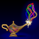coondawg71
Thanks for the comments coondawg71. You are welcome. Michael
Balas